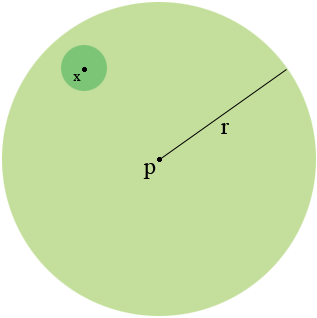SEARCH HOMEMath Central Quandaries & QueriesQuestion from Aqeel, a teacher: Theorem: The open ball is an open set?Hi,

Draw a diagram!My diagram is the open ball of radius $r$ about the point $p.$ Let $x$ be some point in this ball so the distance from $p$ to $x$ is less than $r.$ Construct an open ball about $x$ of radius $\epsilon$ so that the ball remains inside the ball about $p$ of radius $r.$ What can $\epsilon$ be?

Now write a formal proof.

HarleyMath Central is supported by the University of Regina and the Imperial Oil Foundation.